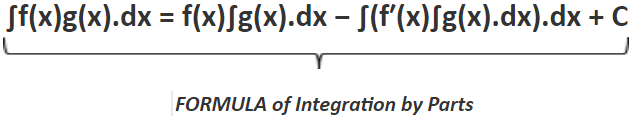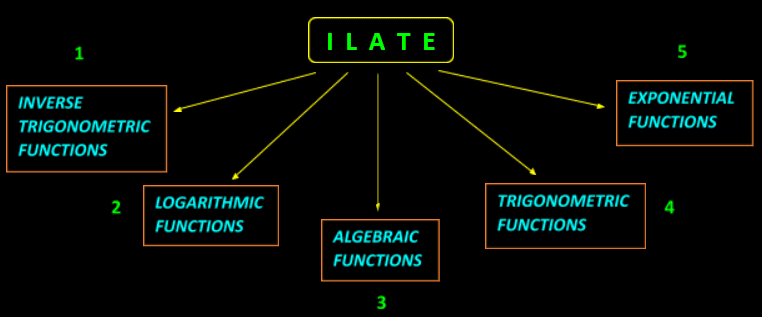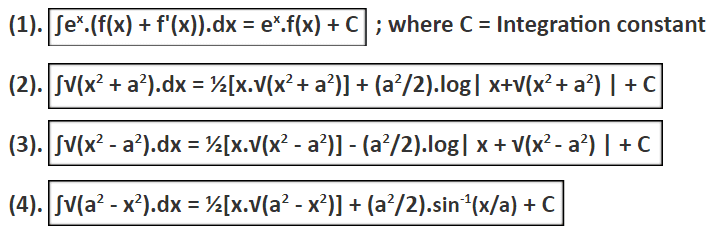Maths
Study Material

# Integration by Parts Formula | ILATE Rule | Examples |

Integration by parts is also called as ‘The Product Rule of Integration’. Here, we shall learn about the concept of integration by parts, its representation along with the formula with some illustrative examples of integration by parts.

4 minutes long
Posted by Kunduz Tutor, 11/10/2021Hesap Oluştur

Got stuck on homework? Get your step-by-step solutions from real tutors in minutes! 24/7. Unlimited.

The formula of integration by parts was proposed by Brook Taylor in 1715. It is a very useful technique to find the integration of the product of two (or more) functions. That’s why integration by parts is also called as ‘The Product Rule of Integration’. Sometimes, it is also referred by the name ‘Partial Integration’.

Moreover, here we shall learn about the concept of integration by parts, its representation along with the formula with some illustrative examples of integration by parts.

Author – Ojasvi Chaplot

## Concept

The product rule of integration states that it is used to find the integral of the product of various types of functions like inverse trigonometric, logarithmic, algebraic, trigonometric and exponential functions.

## Representation

In general, the standard form to represent the functions which are to be integrated is [f(x) and g(x)]. It can also be observed that at some places they are represented as [u and v].

## Integration by Parts Formula

The integration by parts formula is calculated as

Integration of (Function I x Function II) = [ (Function I) x (Integration of Function II) ] – Whole Integration of [ (Differentiation of Function I) x (Integration of Function II) ]Thus, it can be seen that the formula of the product rule of integration is divided in two parts; the first term consists of the function f(x) and the integral of the function g(x) and the second term constitutes of the whole integration of differentiation of the function f(x) and the integral of the function g(x). Along with which, there exists an integration constant also.

## ILATE Rule

While working with the formula of integration by parts, it must be taken care of how to choose the Function I and Function II, i.e. f(x) and g(x) respectively.

To understand in which order, the function must be chosen as f(x) (or u) and as g(x) (or v), there exists a rule called as ‘ILATE’. At some places it is also written as ‘LIATE’.Furthermore, in some exceptional cases, this rule may not be given priority; instead, one must choose it wisely that if that function’s integral exists and is easy to compute then it should be taken as g(x) [i.e. second function] and if it is such a function that it’s differential can be easily obtained then it should be taken as f(x) [i.e. first function].

## Special Case of Integration by Parts Formula

There are some special case formulas for the product rule of integration (integration by parts), which are derived from its standard formula. These can be used directly in between the question instead of showing the whole procedure of their derivation.

Some of the special case formulas are listed below –## Illustrative examples using Formula of Integration by Parts

For Example : Find the integration of xex by using the integration by parts formula.

Solution:

As we know that, ∫f(x)g(x).dx = f(x)∫g(x).dx − ∫(f′(x)∫g(x).dx).dx + C

⇒ ∫xex.dx = ∫x.ex.dx

Further, using ILATE Rule,

⇒ f(x) = x and g(x) = ex

⇒ f’(x) = 1 and ∫g(x).dx = ∫ex.dx = ex.

Then, by using integration by parts,

⇒ ∫xex.dx = xex – ∫1.ex.dx

⇒ ∫xex.dx = xex – ∫ex.dx

⇒ ∫xex.dx = xex – ex

So, ∫xex.dx = ex(x – 1) + C

Therefore, Answer: ∫xex.dx = ex(x – 1) + C ; where C = Integration constant.

For Example : Find the integral of logx by using the integration by parts formula.

Solution:

As we know that, ∫f(x)g(x).dx = f(x)∫g(x).dx − ∫(f′(x)∫g(x).dx).dx + C

⇒ ∫logx.dx = ∫logx.1.dx

Hence, By using ILATE Rule,

Let, f(x) = logx and g(x) = 1

⇒ f’(x) = 1/x and ∫g(x).dx = ∫1.dx = x.

Then, by using integration by parts,

⇒ ∫logx.dx = logx.x – ∫(1/x).x.dx

⇒ ∫logx.dx = x.logx – ∫1.dx

So, ∫logx.dx = x.logx – x + C

Therefore, Answer: ∫logx.dx = x.logx – x + C ; where C = Integration constant.

## Picked For You

Furthermore, these are some topics which might interest you: